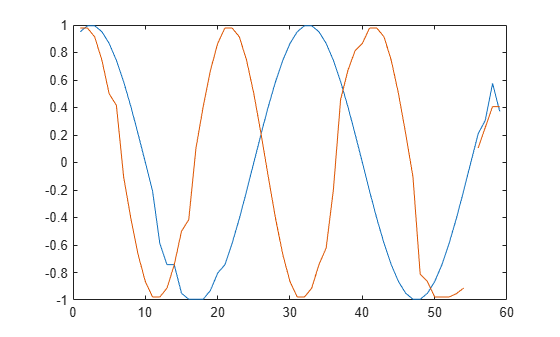# medfilt1

1-D median filtering

## Syntax

``````y = medfilt1(x)``````
``````y = medfilt1(x,n)``````
``````y = medfilt1(x,n,blksz,dim)``````
``y = medfilt1(x,n,[],dim)``
``y = medfilt1(___,nanflag,padding)``

## Description

``````y = medfilt1(x)``` applies a third-order one-dimensional median filter to the input vector, `x`. The function considers the signal to be 0 beyond the endpoints. The output, `y`, has the same length as `x`.```

example

``````y = medfilt1(x,n)``` applies an `n`th-order one-dimensional median filter to `x`.```
``````y = medfilt1(x,n,blksz,dim)``` or `y = medfilt1(x,n,[],dim)` specifies the dimension, `dim`, along which the filter operates. `blksz` is required for backward compatibility and is ignored.```

example

````y = medfilt1(___,nanflag,padding)` specifies how `NaN` values are treated over each segment, using any input arguments from previous syntaxes. This syntax also specifies `padding`, the type of filtering performed at the signal edges.`nanflag` and `padding` can appear anywhere after `x` in the function call.```

## Examples

collapse all

Generate a sinusoidal signal sampled for 1 second at 100 Hz. Add a higher-frequency sinusoid to simulate noise.

```fs = 100; t = 0:1/fs:1; x = sin(2*pi*t*3)+0.25*sin(2*pi*t*40);```

Use a 10th-order median filter to smooth the signal. Plot the result.

```y = medfilt1(x,10); plot(t,x,t,y) legend('Original','Filtered') legend('boxoff')```Generate a two-channel signal consisting of sinusoids of different frequencies. Place spikes in random places. Use NaNs to add missing samples at random. Reset the random number generator for reproducible results. Plot the signal.

```rng('default') n = 59; x = sin(pi./[15 10]'*(1:n)+pi/3)'; spk = randi(2*n,9,1); x(spk) = x(spk)*2; x(randi(2*n,6,1)) = NaN; plot(x)```Filter the signal using `medfilt1` with the default settings. Plot the filtered signal. By default, the filter assigns NaN to the median of any segment with missing samples.

```y = medfilt1(x); plot(y)```Transpose the original signal. Filter it again, specifying that the function work along the rows. Exclude the missing samples when computing the medians. If you leave the second argument empty, then `medfilt1` uses the default filter order of 3.

```y = medfilt1(x',[],[],2,'omitnan'); plot(y')```The function cannot assign a value to the segment that contains only NaNs. Increase the segment length to fix this issue. The change also removes the outlier more thoroughly.

```y = medfilt1(x,4,'omitnan'); plot(y)```The default zero padding results in the function's underestimating the signal values at the edges. Lessen this effect by using decreasing windows to compute the medians at the ends.

```y = medfilt1(x,4,'omitnan','truncate'); plot(y)```## Input Arguments

collapse all

Input signal, specified as a real-valued vector, matrix, or N-D array.

Data Types: `single` | `double`

Order of the one-dimensional median filter, specified as a positive integer scalar.

• When `n` is odd, `y(k)` is the median of `x(k-(n-1)/2:k+(n-1)/2)`.

• When `n` is even, `y(k)` is the median of `x(k-n/2:k+(n/2)-1)`. In this case, `medfilt1` sorts the numbers and takes the average of the two middle elements of the sorted list.

Example: If `n` = 11, then `y(k)` is the median of `x(k-5:k+5)`.

Example: If `n` = 12, then `y(k)` is the median of `x(k-6:k+5)`.

Data Types: `double`

Dimension to filter along, specified as a positive integer scalar. By default, `medfilt1` operates along the first nonsingleton dimension of `x`. In particular, if `x` is a matrix, the function filters its columns so that ```y(:,i) = medfilt1(x(:,i),n)```.

Data Types: `double`

`NaN` condition, specified as `'includenan'` or `'omitnan'`.

• `'includenan'` — Returns the filtered signal so that the median of any segment containing `NaN`s is also `NaN`.

• `'omitnan'` — Returns the filtered signal so that the median of any segment containing `NaN`s is the median of the non-`NaN` values. If all elements of a segment are `NaN`s, the result is `NaN`.

Endpoint filtering, specified as `'zeropad'` or `'truncate'`.

• `'zeropad'` — Considers the signal to be zero beyond the endpoints.

• `'truncate'` — Computes medians of smaller segments as it reaches the signal edges.

## Output Arguments

collapse all

Filtered signal, returned as a real-valued vector, matrix, or N-D array. `y` is the same size as `x`

Data Types: `double`

## Tips

If you have a license for Image Processing Toolbox™ software, you can use the `medfilt2` (Image Processing Toolbox) function to perform two-dimensional median filtering.

 Pratt, William K. Digital Image Processing. 4th Ed. Hoboken, NJ: John Wiley & Sons, 2007.